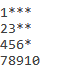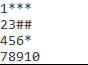# Patterncan you able to give me the solution of these pattern using 2 for loop

this question is a c# language

can you give me the solution

Do you mean you need C# code for InvokeCode?
the following code will work.

``````int c=1;
int i=1;
while( i<=10)
{
for (int j=0; j<4;j++)
{
if (j<c)
{
s+=i.ToString();
i++;
}else{
s+="*";
}
}
s+=Environment.NewLine;
c++;
}
``````

Please set s as string in Argument panel of Invoke code activity.

Regards,

it was right can you give me the solution of this alsousing 2 loop only @Yoichi

Give it a try

``````int c=1;
int i=1;
while( i<=10)
{
for (int j=0; j<4;j++)
{
if (j<c)
{
s+=i.ToString();
i++;
}else{
if (c==2){
s+="#";
}else{
s+="*";
}
}
}
s+=Environment.NewLine;
c++;
}
``````

Regards,

thank you so much it was right code @Yoichi

This topic was automatically closed 3 days after the last reply. New replies are no longer allowed.